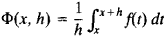# Steklov Function

The following article is from The Great Soviet Encyclopedia (1979). It might be outdated or ideologically biased.

## Steklov Function

a function defined for a given function f(x) by the equalitywhere h is so small that the interval (x, x + h) lies in the domain of definition of f(x).

The Steklov function is used to smooth f(x), since if f(x) is continuous, then Φ(x, h) has one more derivative than f(x, h). Moreover, lim Φ(x, h) = f(x)—that is, the Steklov function can be used to approximate continuous functions by smoother functions. If f(x) is integrable, then Φ(x, h) is continuous. The Steklov function was introduced by V. A. Steklov in 1903 and was used by him to solve many problems in mathematical physics.

The Steklov function can also be defined for more than one variable.